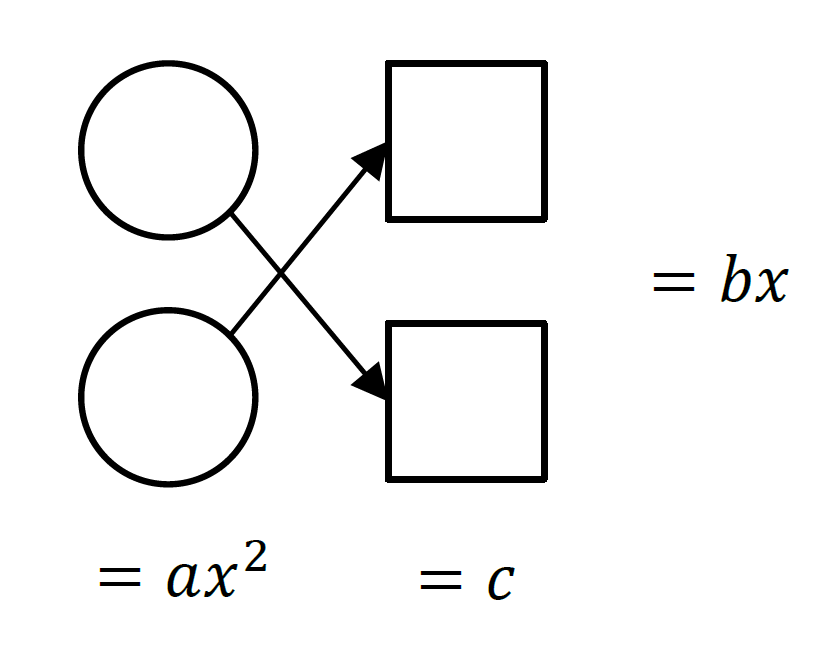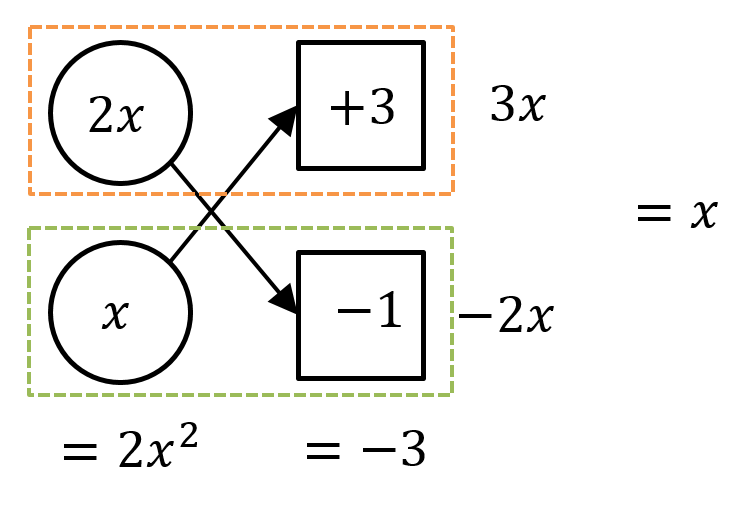# Year 10 Maths Algebra: How To Solve Quadratic Equations [Free Algebra Worksheet]

In this post, we give you a comprehensive cheat-sheet to help you conquer Quadratic Equations for Year 10 Algebra!Being able to solve quadratic equations is an essential skill necessary for a number of topics such as curve sketching, and for finding the minimum or maximum values to solve real-life problems.

## NSW Syllabus Outcomes

Stage 5.3: Solve a wide range of quadratic equations derived from a variety of contexts (ACMNA269)

• solve equations of the form $$ax{^2} + bx + c = 0$$ by factorisation and by ‘completing the square’

• use the quadratic formula $$x= \frac {-b \ \pm \ \sqrt{b{^2} – 4ac}}{2a}$$ to solve quadratic equations

##### Note to students

This page will teach you the methods for factorising quadratic expressions of the form $$ax{^2}+bx+c$$. For all other factorising techniques such as:

• Finding common factors
• Grouping in pairs
• A difference of two squares
• Perfect squares

Please visit Year 9 Maths Algebra – Factorisation Techniques.

## Assumed knowledge

Students should be familiar with basic algebraic techniques including expanding special binomial products and simple arithmetic. Knowledge of factorisation techniques will also be required.

## Content

A quadratic trinomial is an algebraic expression in the form:

$$ax{^2}+bx+c$$

Where:

• $$a$$ is called the quadratic coefficient or the leading coefficient
• $$b$$ is called the linear coefficient
• $$c$$ is called the constant term

A quadratic equation of the form $$ax{^2}+bx+c=0$$ can have up to two real solutions. When we ‘solve’ a quadratic equation, we are looking for the $$x$$-values that make the equation true.

From basic arithmetic, we know that if the product of the two numbers is zero, then at least one of the numbers must be zero.

• In symbols: If $$ab=0$$, then either $$a=0$$ or $$b=0$$  or both $$a$$  and $$b$$ are zero.
• For example: If $$(x+1)(x-2)=0$$ , then either $$(x+1)=0$$, or $$(x-2)=0$$, or both are zero. Hence $$x=-1$$ or $$x=2$$.

To solve a quadratic equation, you must move all the non-zero terms onto the left-hand side first so your equation is in the form $$ax{^2}+bx+c=0$$. Then, try factorising the left-hand side!

### Example

Solve the quadratic equation $$x{^2}-4x=0$$.

### Solution

First, we must factorise the expression on the left-hand side, $$x{^2}-4x$$.

$$x{^2}-4x=x(x-4)$$

Hence we are solving the quadratic equation $$x(x-4)=0$$.

It can be seen that $$x=0$$ or $$(x-4)=0$$  for the equation to be true.

Therefore the solutions are $$x=0$$ or$$x=4$$.

The factorisation used in this example is simple as we only needed to extra a common factor to factorise. However, when you are given a quadratic trinomial to solve, you will need to use the following methods.

## Solving Quadratic Trinomials (which can be factorised)

A monic quadratic trinomial is an expression of the form $$ax{^2}+bx+c$$ where $$x=1$$.

When we expand $$(x+\alpha)(x+\beta)$$, we get $$(x+\alpha)(x+\beta)=x{^2}+(\alpha+\beta)x+\alpha\beta$$.

The coefficient of $$x$$ is $$\alpha+\beta$$ is and the constant term is $$\alpha \beta$$.

Hence, to factorise a monic quadratic trinomial, we must reverse the process by finding two numbers whose:

1. Sum is the linear coefficien
2. Product is the constant term

### Example: Solving Monic Quadratic Equation

Solve the quadratic equation $$x{^2}-2x-8=0$$.

### Solution

Factorising the expression $$x{^2}-2x-8$$ , we must find two numbers whose sum is $$-2$$ and whose product is $$-8$$. The only possible numbers are $$2$$ and $$-4$$.

Therefore $$x{^2}-2x-8=(x+2)(x-4)$$

Hence we are solving the equation $$(x+2)(x-4)=0$$.

It can be seen that $$(x+2)=0$$ or $$(x-4)=0$$.

Therefore the solutions are $$x=-2$$ or $$x=4$$.

## Want to improve your Maths skills?

A non-monic quadratic trinomial is an expression of the form $$ax{^2}+bx+c$$ where $$a \neq 1$$. There are three main strategies for factorising these types of expressions.

## (a) Pairing Method

1. Find two numbers $$p$$ and $$q$$ whose sum is the linear coefficient and whose product is the product of the quadratic coefficient and the constant.

2. Use the two numbers to split the middle term $$bx$$ into the form $$px + qx$$.

3. Complete the factorisation by grouping in pairs.

##### Note To Students

You may revise the ‘grouping in pairs’ technique by visiting Year 9 Maths Algebra – Factorisation Techniques.

### Example: Solving Non-monic Quadratic Equation

Solve quadratic equation $$2x{^2}+3x-5=0$$ .

### Solution

To factorise the expression $$2x{^2}+3x-5$$, we first find the product of the quadratic coefficient and the constant, $$2 \times (-5)= -10$$. Now, we must find two numbers whose sum is $$3$$ and whose product is $$-10$$. The only possible numbers are $$-2$$ and $$5$$.

We use these two numbers to split the middle term and then factorise by grouping in pairs.

$$2x{^2}+3x-5=2x{^2}-2x+5x-5$$

$$= 2x(x-1)+5(x-1)$$

$$=(x-1)(2x+5)$$

Hence, we are solving the equation $$(x-1)(2x+5)=0$$.

Therefore the solutions are $$x=1$$ or $$x= – \frac{5}{2}$$.

## (b) Fraction method

1. Rewrite the trinomial expression as the fraction in the form $$\frac {(ax \: \:)(ax \: \:)}{a}$$.

2. Find two numbers whose sum is the linear coefficient and whose product is the product of the quadratic coefficient and the constant.

3. Place these factors into the empty brackets.

4. Factorise each bracket where possible by extracting common factors.

5. Cancel out common factors.

### Example: Solving Non-monic Quadratic Equation using fraction method

Solve the quadratic equation $$12x^{^{2}}+11x-5=0$$.

### Solution

To factorise the expression $$12x^{2}+11x-5$$ , rewrite the trinomial as a fraction.

$$12x^{2}+11x-5 = \frac{(12x\: \: \: \: \: \: \: \: )(12x\: \: \: \: \: \: \: \: )}{12}$$

Find two numbers whose sum is $$11$$  and whose product is $$-60$$.

The only possible numbers are $$-4$$  and $$15$$ .

Place these factors into the brackets, factorise and cancel.

$$12x^{2}+11x-5 = \frac {(12x-4)(12x+15)}{12}$$

$$= \frac {4(3x-1) \times 3(4x+5)}{12}$$

$$=\frac {12(3x-1)(4x+5)}{12}$$

$$=(3x-1)(4x+5)$$

Hence, we are solving the equation $$(3x-1)(4x+5)=0$$ .

Therefore the solutions are $$x=\frac{1}{3}$$ or $$x=-\frac{5}{4}$$.

## (C) Cross method

Step 1: Set up a workspace as shown:Step 2: Place numbers in the circles such that the product of the circles is the quadratic term.

Step 3: Place numbers in the squares such that the product of the squares is the constant term.

Step 4: The numbers should be chosen such that cross multiplying in the direction of the arrows and adding the two results will give the linear coefficient.

Step 5: Read across each row and combine the circle and the square the find the two factors of the trinomial expression.

### Example: Solving Non-monic Quadratic Equation using cross method

Solve the quadratic equation  $$2x{^2}+x-3=0$$.

### Solution

First, we must factorise the expression $$2x{^2}+x-3$$ .

Setting up the workspace and filling in the circles and squares, we get:The product of the expressions in the circles $$=2x{^2}$$, the quadratic term. The product of the numbers in the squares give $$-3$$, the constant term. Cross-multiplying, we can see that the sum of the results is $$3x+(-2x)=x$$, the linear coefficient.

Hence, $$2x^{2}+x-3 = \color{Orange}{(2x+3)} \color{DarkGreen} {(x-1)}$$ .

We are solving the equation $$(2x+3)(x-1)=0$$

Therefore the solutions are $$x=-\frac{3}{2}$$  or $$x=1$$.

### Note to students

Sometimes it may be necessary to extract a highest common factor (HCF) from the expression before factorising the quadratic trinomial using these strategies. For example, the expression $$6x^{2}+3x-9$$ can be factorised by first removing the HCF of 3.

e.g. $$6x^{2}+3x-9=3(2x^{2}+x-3)= 3(2x+3)(x-1)$$.

## Solving Quadratic Trinomials (which cannot be factorised)

When a quadratic expression cannot be factorised to give rational solutions as we have seen in the examples so far, we need to find other ways to solve the quadratic equation.

### 1. Completing the square

This technique uses the special binomial products referred to as perfect squares:

$$(a\pm b)^{2}= a^{2}\pm2ab+b^{2}$$

To factorise by completing the square:

1. Express the quadratic equation in the form $$x^{2}+kx=m$$  where $$k$$ and $$m$$ are real numbers.
2. Complete the square on the left hand side of the equation by halving the linear coefficient, squaring it, and adding it to both sides of the equation.

### Example: Solving Quadratic Equations using completing the square method

Solve the quadratic equation $$x{^2}-2x-5=0$$.

### Solution

Move the constant over to the right-hand side.

$$x{^2}-2x=5$$

Complete the square on the left-hand side by halving the linear coefficient, squaring it and adding it to both sides of the equation.

$$x^{2}-2x+(\frac{-2}{2})^{2} = 5+ (\frac {-2}{2})^{2}$$

$$x^{2}-2x+1 = 5+1$$

Express the left-hand side as a perfect square and simplify the right-hand side.

$$(x-1){^2}=6$$

Solve the resulting equation by taking the square root of both sides of the equation.

$$x-1= \sqrt{ \pm 6}$$

Therefore, the solutions are $$x= 1 \pm \sqrt {6}$$.

### Note to students

This method of completing the square only works on monic quadratic expressions i.e. quadratic coefficient $$a=1$$. To factorise a non-monic quadratic using this method, start by dividing through by the coefficient of$$x{^2}$$.

For example, $$2x{^2}-4x=5$$  can be rewritten as $$x{^2}-2x= \frac{5}{2}$$ . The method of completing the square can now be applied to this equation.

Applying the method of completing the square to the general quadratic equation $$ax{^2}+bx+c=0$$, a general formula for the solutions of the equation (if they exist) can be derived. This is called the quadratic formula, given by:

$$x= \frac {-b \pm \sqrt {b{^2}-4ac}}{2a}$$

### Note to students

At Matrix, we will provide you with an extensive proof for the derivation of this formula.

Solve the quadratic equation $$x{^2}+6x+2=0$$.

### Solution

Find the values of $$a , b$$ and $$c$$.

$$a=1$$

$$b=6$$

$$c=2$$

Substitute these values into the quadratic formula.

$$x= \frac {-b \pm \sqrt{b{^2}-4ac}}{2a}$$

$$x= \frac {-6 \pm \sqrt{(6){^2}-4(10(2)}}{2(1)}$$

$$= \frac {-6 \pm \sqrt{36-8}}{2}$$

$$= \frac {-6 \pm \sqrt {36-8}}{2}$$

$$= \frac {-6 \pm 2 \sqrt {7}}{2}$$

$$= -3 \pm \sqrt{7}$$

Therefore, the solutions are $$x= -3 \pm \sqrt{7}$$.

## Summary

1. Extract the highest common factor (if any)

2. Factorise the quadratic expression if possible. If it is a non-monic quadratic trinomial, use one of the following factorisation methods:

(a) Pairing method

(b) Fraction method

(c) Cross method

3. If it is not possible to factorise the quadratic expression, use one of the following methods:

(a) Completing the square

## Year 10 Algebra Worksheet – Solving Quadratic Equations

Check your skills with the following 10 exercises!

1.$$2x{^2}-6x=0$$

2. $$x{^2}-3x-4=0$$

3. $$x{^2}+4x-12=0$$

4. $$2x{^2}+5x-3=0$$

5. $$8x{^2}+14x+3=0$$

6. $$-12x{^2}-7x+10=0$$

7. $$x{^2}-4x-10=0$$ (use completing the square method)

8. $$2x{^2}+4x-3=0$$ (use completing the square method)

9. $$5x{^2}-10x+4=0$$ (use the quadratic formula)

10. $$-3x{^2}-5x+6=0$$ (use the quadratic formula)

## Solutions

1. $$x=0$$ or $$x=3$$

2.$$x=-1$$  or $$x=4$$

3.$$x=-6$$  or $$x=2$$

4.$$x= \frac{1}{2}$$  or$$x=-3$$

5. $$x= – \frac{1}{4}$$ or$$x= – \frac{3}{2}$$

6. $$x= \frac {2}{3}$$ or $$x= – \frac{5}{4}$$

7. $$x = 2 \pm \sqrt{14}$$

8. $$x= -1 \pm \sqrt{ \frac {5}{2}}$$

9. $$x= 1 \pm \frac{ \sqrt {5}}{5}$$

10. $$x= \frac {5 \pm \sqrt{97}}{-6}$$

## Do you still need help with Quadratic equations?

Read our Beginner’s Guide to Acing Year 10 Maths article, Part 1: Year 10 Quadratic Equations & Non-Linear Simultaneous Equations!

## Want to take your Year 10 Maths skills next level?